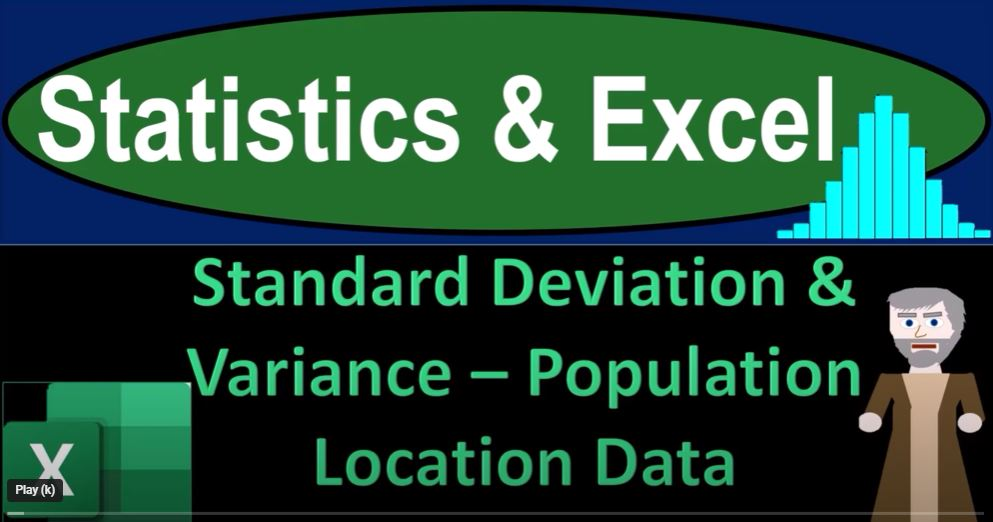## Standard Deviation & Variance – Population Location Data 1452 Statistics & Excel

Welcome to the world of statistics and Excel! In this blog post, we’ll delve into the concepts of standard deviation and variance, particularly when working with population data. So, take a deep breath, hold it in for a few seconds, and get ready for a smooth journey through Excel.

Setting Up in Excel

If you have access to Excel, that’s great. We’ll build our examples using a blank worksheet. If not, don’t worry; you can follow along using your preferred spreadsheet software or even pen and paper. We’ll use three tabs: “Example,” “Practice,” and “Blank Example,” each serving a specific purpose.

Understanding the Data

Our focus today is on understanding how data spreads around the center point, typically the mean or average. To do this, we’ll use standard deviation and variance calculations. Our data consists of two columns: location and population data. You can type this data manually or find datasets on platforms like Kaggle (kaggle.com) for practice.

Excel Formatting

Before diving into calculations, let’s format our Excel worksheet. We’ll make it visually appealing and easier to work with. Formatting includes adjusting cell properties, font styles, and creating a table for our data.

Calculating Basic Statistics

1. Mean (Average): Calculates the average population value.
2. Minimum Value: Finds the smallest population value.
3. Q1 (First Quartile): Identifies the 25th percentile population value.
4. Median (Q2): Determines the middle population value.
5. Q3 (Third Quartile): Identifies the 75th percentile population value.
6. Maximum Value: Finds the largest population value.

Population Standard Deviation and Variance

Now, let’s focus on the new calculations: population standard deviation and population variance. These metrics help us understand how the data is spread out around the mean for the entire population. Note that there are slightly different calculations for samples, which we’ll cover in a future post.

Excel Functions for Calculations

We’ll use Excel functions to calculate these statistics quickly. Here’s how to do it:

• Standard Deviation for Population: Use the formula “=STDEV.P(data range)” to calculate the population standard deviation.
• Variance for Population: Use the formula “=VAR.P(data range)” to calculate the population variance.

Visualizing the Data

To gain a better understanding of the data’s distribution, we can create a histogram in Excel. This graphical representation can help us visualize the shape of the data and its skewness.

Going Deeper with Formulas

For those who want to understand the math behind standard deviation and variance, we’ve included the formulas in our table. These formulas break down the calculations step by step, helping you grasp the concepts more intuitively.

• Difference from Mean: We calculate the difference between each data point and the mean.
• Squared Differences: To eliminate negative values, we square the differences.
• Variance Calculation: We sum the squared differences and divide by the population size.
• Standard Deviation: Finally, we calculate the square root of the variance to get the standard deviation.

By breaking down the calculations, you can gain a deeper insight into how these statistics work and why they are essential in data analysis.

In conclusion, Excel is a powerful tool for performing statistical calculations, including standard deviation and variance. These statistics help us understand the spread of data in a population. Whether you’re a beginner or a seasoned data analyst, mastering these concepts is crucial for making informed decisions based on data.

So, next time you encounter a dataset, remember to take a deep breath, open Excel, and explore the fascinating world of statistics. Happy number crunching!